Study Materials

NCERT Solutions for Class 10th Mathematics

Page 1 of 4

Chapter 2. Polynomials

Exercise 2.1

Chapter 2. Polynomials

Exercise 2.1

Q1. The graphs of y = p(x) are given in Fig. 2.10 below, for some polynomials p(x). Find the number of zeroes of p(x), in each case.Solution(i):

Number of zeroes of p(x) = 0;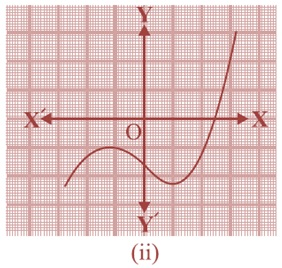Solution(ii):

Number of zeroes of p(x) = 1;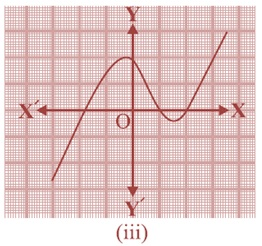Solution (iii):

Number of zeroes of p(x) = 3;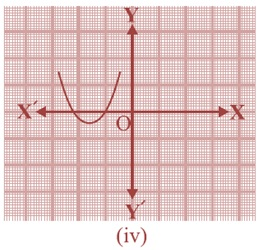Solution (iv):

Number of zeroes of p(x) = 2;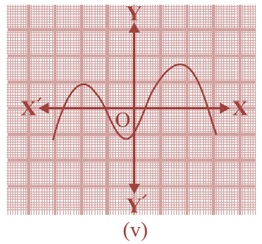Solution (v):

Number of zeroes of p(x) = 4;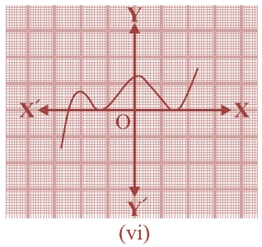Solution (vi):

Number of zeroes of p(x) = 3;

Page 1 of 4

Chapter Contents: